# Tree-Based Machine Learning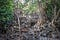Trees & Temple Ruins (Cambodia)

I had always underestimated the power of the decision tree algorithm until I started to write this post. Although the decision tree is algorithmically simple, compared with SVM, Neural Network etc., the decision tree is undoubtedly intuitive and easy to implement. However, simplicity comes with a cost of overfitting (high variance). To overcome overfitting, random forest and boosted trees algorithms come to our rescue.

This is the first part of the decision tree series which covers the following:

Decision Tree — Part 1 (this post)

Random Forest — Part 2 (next post)

Boosted Trees — Part 3 (next post)

For…

# Linear Regression

Kevin Murphy, the author of ‘Machine Learning: A Probabilistic Perspective’, refers to linear regression as a ‘workhorse’ of statistics and supervised machine learning. In its original form, it can model the linear relationship. When augmented with kernels or basis functions, it can capture the non-linear relationship. It can be used as a classifier by replacing Gaussian output with Bernoulli or Multinoulli distribution. Before proceeding, let’s review some notations used here.

y : a scalar output

y: an output vector

x : a feature vector

X: a feature matrix

# 1. General Form

The general form of linear regression is, compactly, given by:

w is…

# Introducing Copula in Monte Carlo Simulation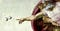Source

In the oil and gas industry, uncertainties are everywhere from the surface to the sub-surface. To embed the uncertainties in any estimation, probabilistic approaches are required.

One of the simple cases is a volumetric estimation. The formulas to estimate hydrocarbon (oil/gas) initially in place (HCIIP) are given below:

# Explicit Recommender System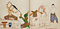Source

Recently, I have been quite fascinated by the recommender system using matrix factorization. There exist a number of existing tools/libraries such as Suprise, Implicit and PySpark for training recommender systems. However, those libraries are not meant to be used by anyone; they do assume a certain level of subject matter understanding. That is precisely why I wrote this post. In the below sections, I will discuss recommender systems and implement a simple one using PyTorch.

# Recommender System

Recommender systems aim to predict users’ interests and recommend products that are fascinating for them. Such knowledge is important for e-commerces and retailers to run…

# Gradient Descent

Gradient descent algorithm and its variants ( Adam, SGD etc. ) have become very popular training (optimisation) algorithm in many machine learning applications. Optimisation algorithms can be informally grouped into two categories — gradient-based and gradient-free(ex. particle swarm, genetic algorithm etc.). As you can guess, gradient descent is a gradient-based algorithm. Why gradient is important in training machine learning?

The objective of training a machine learning model is to minimize the loss or error between ground truths and predictions by changing the trainable parameters. And gradient, which is the extension of derivative in multi-dimensional space, tells the direction along which…

# Convolutional Neural Network — An Informal Introduction (Part-1)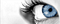http://introtodeeplearning.com/

The convolutional neural network aka CNN has become a de facto practice for image classification and detection. Machine learning frameworks like PyTorch and TensorFlow provide convenient means to rapidly construct a CNN. Therefore, it is unlikely that we need to start a CNN project from scratch totally since there exist published CNN architectures like (AlextNet, VGG16, Darknet19, etc.) which can be readily adapted for image classification and detection projects. Having a good understanding of how a CNN works, however, is of importance when we need to work with any image classification and detection task.

# Introduction to Principal Component Analysis

By In Visal, Yin Seng, Choung Chamnab & Buoy Rina — this article was presented to ‘Facebook Developer Circle: Phnom Penh’ group on 20th July 2019. Here is the original slide pack — https://bit.ly/2Z1pyAb

# Why PCA?

According to DataCamp, PCA can be viewed in the following ways:

• One of the more-useful methods from applied linear algebra
• Non-parametric way of extracting meaningful information from confusing data sets
• Uncovers hidden, low-dimensional structures that underlie your data
• These structures are more-easily visualized and are often interpretable to content experts

# Formal Definition

Accordingly to Wikipedia:

“Principal component analysis (PCA) is a statistical procedure that uses an orthogonal transformation…

# Understanding Gaussian Classifier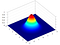Normal Distribution (Wikipedia)

Experience is a comb which nature gives us when we are bald. ~Proverb

# Overview

The normal distribution is perhaps one of the commonly used statistical distribution functions. It is basically everywhere. Chances are that we have already used it directly or indirectly. In this article, we discuss univariate & multivariate normal distribution, and how we can derive a generative (more on that later) Gaussian classifier using Bayes’ theorem. In my opinion, classifiers like Gaussian are simple yet intuitive and interpretable.

The main reference for this article is Machine Learning: A Probabilistic Perspective by Kevin P. Murphy

# Normal/Gaussian Distribution

In real life, there are…## Rina Buoy

An applied NLP researcher at Techo Startup Center (TSC)